# chessian

Hessian of a multivariate, scalar-valued function using the central difference approximation.

## Syntax

H = chessian(f,x0)
H = chessian(f,x0,h)


## Description

H = chessian(f,x0) numerically evaluates the Hessian of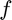with respect to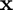at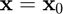using the central difference approximation with a default relative step size of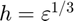, where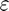is the machine zero.

H = chessian(f,x0,h) numerically evaluates the Hessian ofwith respect toatusing the central difference approximation with a user-specified relative step size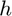.

## Input/Output Parameters

 Variable Symbol Description Format Input f multivariate, scalar-valued function () 1×1function_handle x0 evaluation point n×1double h (OPTIONAL) relative step size 1×1double Output H Hessian of with respect to , evaluated at n×ndouble

## Note

• This function requires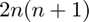evaluations of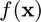.

## Example

Approximate the Hessian of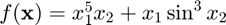at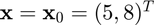using the chessian function, and compare the result to the true result of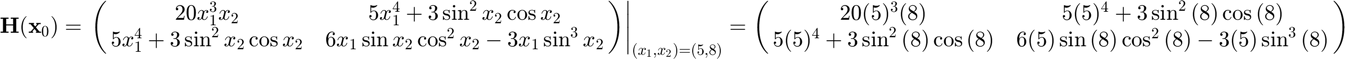Approximating the Hessian,

f = @(x) x(1)^5*x(2)+x(1)*sin(x(2))^3;
x0 = [5;8];
H = chessian(f,x0)

H =

1.0e+04 *

2.0000    0.3125
0.3125   -0.0014



Calculating the error,

error = H-[20*5^3*8,5*5^4+3*sin(8)^2*cos(8);5*5^4+3*sin(8)^2*cos(8),...
6*5*sin(8)*cos(8)^2-3*5*sin(8)^3]

error =

1.0e-03 *

0.0072    0.2459
0.2459    0.1293